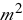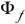# Day 128

## Early Attempts at Generating an Electric Current

• Michael Faraday tried to generate an electric current from a magnetic field, on the heels of Oersted’s discovery that an electric current produced a magnetic field.
• Joseph Henry was simultaneously experimenting and generating similar results.

• A primary coil was wrapped around an iron ring; a battery supplied the current and a switch was in the circuit.
• A secondary coil was wrapped on the other side of the iron ring and was attached to a galvanometer.

• When the switch is closed (primary coil), a momentary deflection is seen on the galvanometer attached to the secondary coil.
• When the switch is opened, a momentary deflection is seen in the galvanometer in the opposite direction.
• When there is a steady current in the primary circuit, the galvanometer reads zero.

• An electric current can be produced by a changing magnetic field; therefore, an induced emf (voltage) is produced in the secondary circuit by the changing magnetic field.

## Magnetic Flux

• The emf produced by the changing magnetic field is actually induced by a change in the magnetic flux Magnetic flux – the product of the cross sectional area of the wire loop and the strength of the magnetic field perpendicular to the wire loop – designated by the Greek letter Φ.

## Magnetic Flux Equation

Φ = BA cos θ

• Unit of flux is the Weber (Wb)
• B (magnetic field) is in Teslas (Wb/)
• A (area of wire) is in• If B isto the wire loop, Θ =, Φ is maximum
• If B is || to the wire loop, Θ = 90°, Φ is zero

## Magnetic Flux Hints

• θ is the angle between the magnetic field and the normal () to the loop.
• The value of Φ is proportional to the number of magnetic field lines passing through the loop.
• The more lines that pass through (cut through) the loop when it isto the magnetic field, the greater the flux!

## Faraday’s Law of Magnetic Induction (Conceptual)

• A current is set up in a circuit as long as there is relative motion between the magnet and the loop.
• The instantaneous emf induced in a circuit = the rate of change of magnetic flux through the circuit.

## Faraday’s Law of Magnetic Induction (Equation)

ε= -N (ΔΦ/Δt)

ε is the induced emf in Volts

• N is the # of loops in the circuit
• ΔΦ is the change in mag flux (–), in Wb
• Δt is the change in time, in sec
• (-) is the polarity of the induced emf

## Try This to Simulate Flux

• If you place a loop of masking tape and a pencil at right angles () to each other, this simulates an angle of 0° with the normal and therefore max flux.
• If you turn the pencil so that it is || to the tape, it cannot cut through the loop, the angle between the normal to the loop and the pencil is 90° = min flux.

## Lenz’s Law

• States that the polarity (sign) of the induced emf is such that it produces a current whose magnetic field opposes the change in magnetic flux through the loop.
• “GFI” – ground fault indicator – is a good example of an application of Lenz’s Law

## Generators

• The principle of electromagnetic induction is the basis for a generator. Generators converts mechanical to electrical energy.
• Rotating a loop of wire on an axle in a magnetic field generates a current in the loop.

(source)